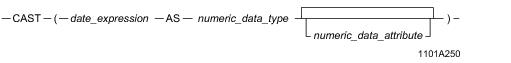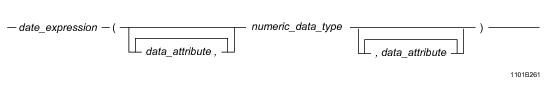# DATE-to-Numeric Conversion - Teradata Database

## Teradata Database SQL Functions, Operators, Expressions, and Predicates

prodname
vrm_release
15.00
category
Programming Reference
featnum
B035-1145-015K

## DATE-to-Numeric Conversion

### Introduction

DATE data may be converted to the following numeric types:

• BYTEINT
• SMALLINT
• INTEGER
• BIGINT
• DECIMAL(n,m)
• NUMBER
• FLOAT
• ### CAST Syntaxwhere:

 Syntax element … Specifies … date_expression a date expression to be converted. numeric_data_attribute one of the following optional data attributes: FORMAT NAMED TITLE

### ANSI Compliance

This is ANSI SQL:2011 compliant.

As an extension to ANSI, CAST permits the use of numeric data attribute phrases.where:

 Syntax element … Specifies … date_expression a date expression to be converted. data_attribute one of the following optional data attributes: FORMAT NAMED TITLE numeric_data_type the target numeric type to which the date expression is to be converted.

### ANSI Compliance

This is a Teradata extension to the ANSI SQL:2011 standard.

### Usage Notes

When a date is converted to a numeric, the value returned is the integer value for the internal stored date, which is encoded using the following formula:

`   (year - 1900) * 10000 + (month * 100) + day`

Allowable date values range from AD January 1, 0001 to AD December 31, 9999.

For example, December 31, 1985 would be stored as the integer 851231; July 4, 1776 stored as -1239296; and March 30, 2041 stored as 1410330.

Conversion of DATE to DECIMAL(n,m) where the number of digits (n) is too small generates a numeric overflow error. Conversion of DATE to BYTEINT or SMALLINT generates a numeric overflow error if the value returned is outside the range of values that the data type can represent.

No error is generated on conversion of DATE to INTEGER or FLOAT.

### FORMAT Phrase

A FORMAT phrase in DATE to numeric conversion may only contain the 9 or Z formatting character. For example:

`   SELECT CAST (DATE '2007-12-31' AS INTEGER FORMAT '9999999');`

### Implicit DATE-to-Numeric Conversion

Teradata Database performs implicit DATE-to-numeric type conversion when you assign a DATE type to a numeric type, compare a DATE type and numeric type, or pass a DATE type to a system function that takes a numeric type.

### Example

The following example converts DATE data in the dob column of the employee table to a numeric format.

Note that the best practice is to define date data as a DATE type; do not define date data as a numeric type.

To change the display from date format to integer format, change the statement to:

`   SELECT name, dob (INTEGER) `
`   FROM employee `
`   WHERE dob BETWEEN 380307 AND 420825 `
`   ORDER BY dob ;`

or

`   SELECT name, CAST (dob AS INTEGER) `
`   FROM employee `
`   WHERE dob BETWEEN 380307 AND 420825 `
`   ORDER BY dob ;`

and the display becomes:

`   Name             DOB`
`   ----------    ------`
`   Inglis C      380307`
`   Peterson J    420327`

### Related Topics

For details on data types and data attributes, see SQL Data Types and Literals.